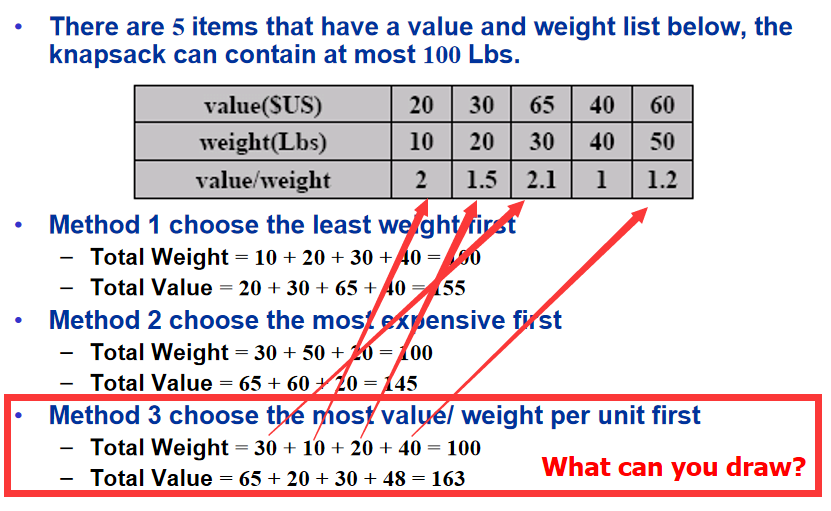# 一、背包问题

## 1.描述

• n：物品的个数
• i：物品的序号
• v i v_i ：某物品的价值value
• w i w_i ：某物品的重量weight
• W：背包的最大承重量

• x i x_i ：表示该物品装入背包多少。在0-1背包问题中，0（不拿）或1（拿走整件）；在分数背包问题中，是个浮点数 [ 0.0 , 1.0 ] [0.0, 1.0] 0.0（不拿）或0.x（拿走部分）或1.0（拿走整件）

## 2.难度划分

• 简单：分数背包问题(Fractional knapsack)
• 难：0-1背包问题(0-1 knapsack)

# 二、简单：分数背包问题(Fractional knapsack)

## 1.思路## 2.伪代码

// v是vi数组，每个物品的价值
// w是wi数组，每个物品的重量
// W是背包的最大负重
// x是xi数组，每个物品装入背包的多少
// n是物品的数量
// 注意：对v数组和w数组有要求，按照性价比降序排列物品，即v(i)/w(i) ≥ v(i+1)/w(i+1)
// 初始化都为0，哪个都不装入
x ← 0
// 背包的剩余容量，开始什么都不装
c ← W
// 遍历每个物品
for i ← 1 to n
// 如果物品的重量小于等于背包剩余容量，则可装入
do	if w(i) ≤ c
// 装入整件物品
then	x(i) ← 1
// 背包剩余容量变少
c ← c - w(i)
// 如果装不进去，那么退出for循环
else	exit

// i ≤ n表示exit退出for循环（注意：for成功遍历完每个物品后，i++的最后结果是i=n+1）
// 如果i ≤ n，说明两种情况，一种是整件物品装不进去但可以装进部分，另一种是背包剩余空间用尽了
if i ≤ n
// 装入该物品的部分：剩余空间/物品总重
// 第二种情况进入没事，因为c = 0 时,x(i) = 0
// 不存在c>=w(i)的情况，要不然就不会exit退出for循环
then	x(i) ← c/w(i)
return x


## 3.c++实现

#include <iostream>
#include <string.h>
using namespace std;

// 物品的个数
#define n 5

// 调整为性价比降序
void costPerformance_insertSort(double v[n], double w[n])
{

// int c[n] = {};
// for(int i = 1;i<n;i++)
// 遍历第二个到最后一个，为A[i]，表示要插入排序好部分的元素。
// 因为第一个不用比较，所以从第二个开始
for (int i = 1; i < n; i++)
{

// 当前待插入的元素
double key_v = v[i];
double key_w = w[i];
// 将A[i]插入到已经排序好的部分，就是A[i]前面的部分A[1...i-1]
// 倒着看前面的，确定要插入的位置
int j = i - 1;
// 在不越界时，并且因为是降序所以是当前一个元素更小时继续
while (j >= 0 && v[j] / w[j] < key_v / key_w)
{

// 前一个元素后移
v[j + 1] = v[j];
w[j + 1] = w[j];
// 再往前面一个
j--;
}
// 此时才正式插入元素
// 因为退出while循环时是A[j]>=key，所以插入到A[j]后面A[j+1]
v[j + 1] = key_v;
w[j + 1] = key_w;
}
}

void gready_fractional_knapsack(double v[n], double w[n], double W, double x[n])
{

// 初始化都为0，哪个都不装入
memset(x, 0, sizeof(x));
// 背包的剩余容量，开始什么都不装
double c = W;
// 遍历每个物品
int i;
for (i = 0; i < n; i++)
{

// 如果物品的重量小于等于背包剩余容量，则可装入
if (w[i] <= c)
{

// 装入整件物品
x[i] = 1;
// 背包剩余容量变少
c -= w[i];
}
// 如果装不进去，那么退出for循环
else
{

break;
}
}
// i ≤ n表示exit退出for循环（注意：for成功遍历完每个物品后，i++的最后结果是i=n+1）
// 如果i ≤ n，说明两种情况，一种是整件物品装不进去但可以装进部分，另一种是背包剩余空间用尽了
if (i <= n)
{

// 装入该物品的部分：剩余空间/物品总重
// 第二种情况进入没事，因为c = 0 时,x(i) = 0
// 不存在c>=w(i)的情况，要不然就不会exit退出for循环
x[i] = c / w[i];
}
}
int main()
{

// 因为算性价比是小数，用double方便
// 某物品的价值value
double v[n] = {
20, 30, 65, 40, 60};
// 某物品的重量weight
double w[n] = {
10, 20, 30, 40, 50};
// 背包的最大承重量
double W = 100;
// 该物品装入背包多少
double x[n] = {
};

// 调整为性价比降序
costPerformance_insertSort(v, w);

// 背包算法

// 总重
double my_weight = 0;
// 总价值
double my_value = 0;

// 输出
for (int i = 0; i < n; i++)
{

my_weight += w[i] * x[i];
my_value += v[i] * x[i];
cout << x[i] << " ";
}
cout << endl;

printf("[my_weight]%lf\n", my_weight);
printf("[my_value]%lf\n", my_value);

return 0;
}


# 三、难：0-1背包问题(0-1 knapsack)


import java.util.*;

public class Knapsack {

class Commodity implements Comparable
{

int num;
int values;
int weight;

public Commodity(int num, int values, int weight)
{

this.values = values;
this.weight = weight;
this.num = num;
}

@Override
public int compareTo(Object o) {
//����
double vdw1 = (double)this.values/this.weight;
double vdw2 = (double)((Commodity)o).values/((Commodity)o).weight;
if(vdw1 > vdw2) return -1;
else if(vdw1 == vdw2) return 0;
else return  1;
}
}

public static void one_zero_knapsack(Commodity[] commodities, int bag, boolean[] x)
{

int n = commodities.length;
boolean[][] memory = new boolean[commodities.length+1][bag+1];
int[][] c = new int[commodities.length+1][bag+1];
caculate_c(commodities, c, memory, x, commodities.length,bag);
}

static void caculate_c(Commodity[] commodities, int[][] c, boolean[][] memory, boolean[] x, int i, int bag)
{

if(i == 0 || bag ==0)
{

c[i][bag] = 0;
memory[i][bag] = true;
}

else if(commodities[i-1].weight > bag)
{

if(!memory[i-1][bag])
{

caculate_c(commodities, c, memory, x, i-1, bag);
}
c[i][bag] = c[i-1][bag];
memory[i][bag] = true;
}
else if(i>0 && bag>=commodities[i-1].weight)
{

if(!memory[i-1][bag-commodities[i-1].weight])
{

caculate_c(commodities, c, memory, x, i-1, bag-commodities[i-1].weight);
}

if(!memory[i-1][bag])
{

caculate_c(commodities, c, memory, x, i-1, bag);
}

int value1 = c[i-1][bag-commodities[i-1].weight] + commodities[i-1].values;
int value2 = c[i-1][bag];
if(value1 > value2)
{

x[commodities[i-1].num] = true;
c[i][bag] = value1;
memory[i][bag] = true;
}
else
{

c[i][bag] = value2;
memory[i][bag] = true;
}
}

}

public static void fractional_knapsack(Commodity[] commodities, int bag, double[] x)
{

Arrays.sort(commodities);
int n = commodities.length;
for(int i=0; i<n; ++i)
{

if(bag - commodities[i].weight >= 0)
{

bag -= commodities[i].weight;
x[commodities[i].num] = 1;
}
else
{

x[commodities[i].num] = (double)bag/commodities[i].weight;
break;
}
}
}

/**
* @param args
*/
public static void main(String[] args) {

Knapsack ks = new Knapsack();
Commodity[] commodities = {
ks.new Commodity(0,20,10),
ks.new Commodity(1,30,20),
ks.new Commodity(2,65,30),
ks.new Commodity(3,40,40),
ks.new Commodity(4,60,50)};
//Arrays.sort(commodities);
int bag = 100;
//0-1 knapsack
boolean[] one_zero = new boolean[commodities.length];
for(int i=0; i<commodities.length; ++i)
{

one_zero[i] = false;
}
Knapsack.one_zero_knapsack(commodities, bag, one_zero);
System.out.println("0-1 knapsack:");
for(int i=0; i<commodities.length; ++i)
{

System.out.println(one_zero[i]);
}
//fractional knapsack
double[] fra  = new double[commodities.length];
Knapsack.fractional_knapsack(commodities, bag, fra);
System.out.println("fractional knapsack:");
for(int i=0; i<commodities.length; ++i)
{

System.out.println(fra[i]);
}

}

}


### java常用的搜索引擎_各大搜索引擎，Java面试题（集合+并发+调优+微服务）_王诗洋的博客-程序员宅基地

Synchronized 用过吗，其原理是什么？你刚才提到获取对象的锁，这个“锁”到底是什么？如何确定对象的锁？什么是可重入性，为什么说 Synchronized 是可重入锁？JVM 对 Java 的原生锁做了哪些优化？48为什么说 Synchronized 是非公平锁？49什么是锁消除和锁粗化？49为什么说 Synchronized 是一个悲观锁？乐观锁的实现原理又是什么？什么是 CAS，它有什...

### matlab 代码 经典例题,MATLAB程序设计及经典例题解析3_天下事一点通的博客-程序员宅基地

MATLAB程序设计用MATLAB语言编写的程序，称为M文件。M文件可以根据调用方式的不同分为两类：命令文件(Script File)和函数文件(Function File)。例3-1 分别建立命令文件和函数文件，将华氏温度f转换为摄氏温度c。程序1：首先建立命令文件并以文件名f2c.m存盘。clear; %清除工作空间中的变量f=input('Input Fahrenheit temperatu...

### windows下常用cmd命令_无效格式的博客-程序员宅基地

windows下常用cmd命令新建文件: type nul &gt; test.txt向文件中写入文字：echo ‘Hello, world’ &gt; test.txt重命名文件：rename test.txt test2.txt复制文件：copy test2.txt test3.txt删除文件：del test2.txt新建文件夹命令：md(或者 mkdir) test删除文件夹命令（文件夹需为空): rd test强制删除文件夹（提示信息）：rd /s test强制删除文件夹（不提

### c++学习之：根据GetLastError()返回值获取错误信息_weixin_33907511的博客-程序员宅基地

VC中GetLastError()获取错误信息的使用在VC中编写应用程序时，经常需要涉及到错误处理问题。许多函数调用只用TRUE和FALSE来表明函数的运行结果。一旦出现错误，MSDN中往往会指出请用GetLastError()函数来获得错误原因。     可问题是，GetLastError()返回的只是一个双字节数值（DWORD）。OH，MY GOD！目前Win32的出错编号已经从0排到11...

### .net core MVC中级教程（三）_weixin_30279315的博客-程序员宅基地

1、对昨天主页不能显示添加的新成员信息bug进行修改2、运用EFCore将其数据写入数据库中1、对昨天主页不能显示添加的新成员信息bug进行修改修改下生命周期，在运行Transient：每一次GetService都会创建一个新的实例Scoped：在同一个Scope内只初始化一个实例 ，可以理解为（ 每一个request级别只创建一个实例，同...

### jquery ajax方法兼容ie9,Jquery AJAX not working in IE9_姜兴的博客-程序员宅基地

I am writing an ajax web application but for whatever reason when I perform a GET against an internal data service in Internet Explorer 9 (IE9) it does not work. This same call works perfectly fine in...

### Java中int的取值范围_第十七签的博客-程序员宅基地

int型的数据字节长度是4，一共是32位，第一位用于表示正负号，其他位的数字组成它所代表的值，例如7的二进制表示形式为： 00000000 00000000 00000000 00000111 当表示负数的时候，则采用的是补码的形式，第一位是1，例如-7的二进制表示为： 11111111 11111111 11111111 11111001 int型数据的取值范围：-2^31—-2^31-1